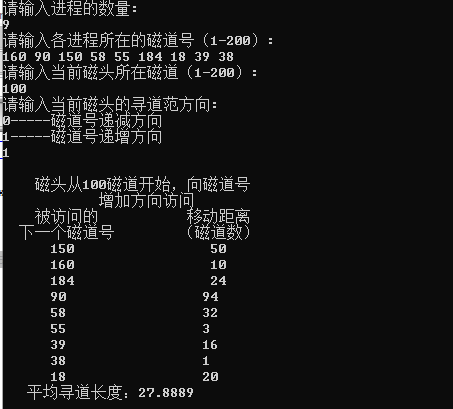• 磁盘寻道算法 电梯调度算法 C++实现 #include<iostream> using namespace std; #include<vector> #include<algorithm> compute(vector<int> v,int x,int d) { double w=1; sort(v.begin()...
磁盘寻道算法 电梯调度算法 C++实现
#include<iostream>
using namespace std;
#include<vector>
#include<algorithm>
compute(vector<int> v,int x,int d)
{
double w=1;
sort(v.begin(),v.end());
if(d==1){
cout<<endl<<"    磁头从"<<x<<"磁道开始，向磁道号"<<endl;
cout<<"            增加方向访问"<<endl;
cout<<"    被访问的           移动距离    "<<endl;
cout<<"  下一个磁道号        （磁道数）   "<<endl;
int i;
for(i=0;i<v.size();i++){
if(x<v[i])
break;
}
int g=i,s=0;;
int m=v[i]-x,x1=x;
for(int j=0;j<v.size();j++){
m=abs(v[i]-x1);
cout<<"      "<<v[i]<<"                 "<<m<<endl;
w+=m;
x1=v[i];
if(i==v.size()-1){
i=g;s=1;
}
if(s==0){
i++;
}
else{
i--;
}
}
}
else{
cout<<endl<<"    磁头从"<<x<<"磁道开始，向磁道号"<<endl;
cout<<"           减少方向访问"<<endl;
cout<<"    被访问的           移动距离    "<<endl;
cout<<"  下一个磁道号        （磁道数）   "<<endl;
int i;
for(i=0;i<v.size();i++){
if(x<v[i+1])
break;
}
int g=i,s=0;;
int m=v[i]-x,x1=x;
for(int j=0;j<v.size();j++){
m=abs(v[i]-x1);
cout<<"      "<<v[i]<<"                 "<<m<<endl;
w+=m;
x1=v[i];
if(i==0){
i=g;s=1;
}
if(s==0){
i--;
}
else{
i++;
}
}
}
cout<<"   平均寻道长度："<<w/v.size()<<endl;
}
int main()
{
vector<int> v;
int n,x,d;
cout<<"请输入进程的数量:"<<endl;
cin>>n;
cout<<"请输入各进程所在的磁道号（1-200）:"<<endl;
for(int i=0;i<n;i++){
int o;cin>>o;
v.push_back(o);
}
cout<<"请输入当前磁头所在磁道（1-200）:"<<endl;
cin>>x;
cout<<"请输入当前磁头的寻道范方向:"<<endl;
cout<<"0-----磁道号递减方向"<<endl;
cout<<"1-----磁道号递增方向"<<endl;
cin>>d;
compute(v,x,d);
}
/*
9
160 90 150 58 55 184 18 39 38
100
1

9
150 160 184 90 58 55 39 38 18
100
0

*/展开全文c++ 操作系统
• 操作系统课程设计，磁盘寻道算法。做了扫描和循环扫描算法。分别用数组和链表方式进行了测试。并比较了时间效率。
• 操作系统中的，4种寻道算法。FCFS(先来先服务) SSTF(最短寻道时间) SCAN(扫描算法) CSCAN(循环扫描法)
• #include <stdio.h> #include <stdlib.h> int tracks;//track number void Init(int start,int num);//initialize track sequence void FCFS(int start,int num);//First come first serve algori...
#include <stdio.h>
#include <stdlib.h>
int tracks;//track number

void Init(int start,int num);//initialize track sequence
void FCFS(int start,int num);//First come first serve algorithm
void SSTF(int start,int num);//Shortest seek time first algorithm

void Init(int start,int num)
{
int i;
printf("----------Input Track Number---------\n");
for(i=0;i<num;i++)
{
printf("Please enter track number %d : ",i+1);
scanf("%d",&tracks[i]);
}

}
void FCFS(int start,int num)
{
double sum=0;
int temp,i;
printf("==========================\n");
printf("Starting from track number %d\n",start);
printf("Next track number:\t seek length:\n");
for(i=0;i<num;i++)
{
temp=abs(start-tracks[i]);
printf("%d\t\t\t %d\n",tracks[i],temp);
sum+=temp;
start=tracks[i];
}
printf("Average seek length:%.3lf\n",sum/num);
printf("==========================\n");
}
void SSTF(int start,int num)
{
int i,j,temp,min,mint;
bool table;
double sum=0;
for(i=0;i<num;i++)
{
table[i]=0;
}
printf("==========================\n");
printf("Starting from track number %d\n",start);
printf("Next track number:\t seek length:\n");
for(i=0;i<num;i++)
{
min=-1;
for(j=0;j<num;j++)
{
if(!table[j])
{
temp=abs(start-tracks[j]);
if(min==-1||min>temp)
{
min=temp;
mint=j;
}
}
}
printf("%d\t\t\t %d\n",tracks[mint],min);
start=tracks[mint];
table[mint]=1;
sum+=min;
}
printf("Average seek length:%.3lf\n",sum/num);
printf("==========================\n");
}

int main()
{
int start;//Initial track number
int num;//visit times
int choose;
printf("\t\t\t Disk Scheduling Algorithms\n");

do
{
printf("Please enter the initial track number(0~200):");
scanf("%d",&start);
}while(start<0||start>200);
do
{
printf("Please enter the number of disk accesses(1~10):");
scanf("%d",&num);
}while(num<1||num>10);

Init(start,num);
do
{
do
{
printf("1.FCFS\n");
printf("2.SSTF\n");
printf("3.exit\n");
printf("Please select:");
scanf("%d",&choose);
}while(choose<1||choose>5);
switch(choose)
{
case 1:
FCFS(start,num);
break;
case 2:
SSTF(start,num);
break;
case 3: exit(0);
}
}while(choose!=5);
return 0;
}




展开全文• printf("请选择：\n1、SSTF（最短寻道优先算法）\n2、CSCAN（循环扫描算法）\n"); printf("3、SCAN（扫描算法）\n4、重新产生一组新的序列\n5、结束\n\n"); char c; while(c=getchar(),c!='5'){ switch(c){ ...
#include<stdio.h>
#include<time.h>
#include<stdlib.h>
#include<string.h>
#include<malloc.h>
#include<math.h>
#define MAX 100 //磁道范围
#define N 20   //序列数目

int list[MAX];  //存放随机产生的序列
int visit[MAX]; //标记数组
int location;//初始磁头位置
int closedistant; //保存其他磁道与当前磁头所在磁道的最短距离

//按磁道从小到大排序
void sort()
{
int i;
int j;
int t;
for(i=0;i<N-1;i++){
for(j=i+1;j<N;j++){
if(list[j]<list[i]){
t=list[i];
list[i]=list[j];
list[j]=t;
}
}
}
}

//随机产生磁头初始位置和访问序列
void CreateList()
{
int j;
int i;
location=rand()%MAX+1;//随机产生初始磁头位置
printf("当前磁头位置：%d\n\n",location);
printf("请求访问的磁道号序列：\n");
for(i=0;i<N;i++){           //随机产生请求磁道号序列
list[i]=rand()%MAX+1;
printf("%d ",list[i]);
}
printf("\n\n");
}

//最短寻道优先算法
void SSTF()
{
int i;
int j;
int k;//记录最近的磁道号
int loca;
int move;
int shortest;  //记录最短的距离
int after[MAX];
memset(visit,1,sizeof(visit));
move=0;
loca=location;
for(i=0;i<N;i++){
shortest=MAX;
for(j=0;j<N;j++){//有更近磁道更新
if(visit[j]){
if(fabs(loca-list[j])<shortest){
shortest=fabs(loca-list[j]);
k=j;
}
}
}
move=move+shortest;  //记录移动的距离
loca=list[k];  //跟踪当前磁头所在位置
visit[k]=0;  //标记已访问
after[i]=list[k];  //记录路径
}
printf("\n采用SSTF算法后的寻道序列为：\n");
for(i=0;i<N-1;i++){
printf("%d->",after[i]);
}
printf("%d\n\n",after[i]);
printf("所有进程运行后磁头运行的总磁道数为：%d\n\n",move);
printf("平均寻道时间为：%.2lf\n\n",move*1.0/N);
printf("\n-------------------------------------------------------\n\n");
}

//循环扫描算法
void CSCAN()
{
int i;
int j;
int move;
int loca;
int after[MAX];
i=0;
loca=location;
for(i=0;i<N;i++){      //找到磁头在序列中的位置
if(list[i]>=loca){
break;
}
}
move=0;
for(j=0;j<N;i++,j++){
if(i==N){         //形成头尾相连
i=0;
}
move=move+fabs(list[i]-loca);  //记录移动的距离
loca=list[i];    //记录当前磁头所在位置
after[j]=list[i];  //记录路径
}
printf("\n本算法规定磁头自里向外移动\n\n");
printf("采用CSCAN算法后的寻道序列为：\n");
for(i=0;i<N-1;i++){
printf("%d->",after[i]);
}
printf("%d\n\n",after[i]);
printf("所有进程运行后磁头运行的总磁道数为：%d\n\n",move);
printf("平均寻道时间为：%.2lf\n\n",move*1.0/N);
printf("\n-------------------------------------------------------\n\n");
}

//扫描算法
void SCAN()
{
int i;
int j;
int k;
int move;
int loca;
int after[MAX];
loca=location;
i=0;
for(i=0;i<N;i++){   //找到当前磁头所在位置
if(list[i]>=loca){
break;
}
}
j=i-1;
k=0;
move=0;
while(i<N){//先自里向外
move=move+fabs(list[i]-loca);//记录移动的距离
loca=list[i]; //记录当前磁头位置
after[k++]=list[i];//记录路径
i++;
}
while(j>=0){//后自外向里 
move=move+fabs(list[j]-loca);//记录移动的距离
loca=list[j]; //记录当前磁头位置
after[k++]=list[j];//记录路径
j--;
}
printf("\n本算法规定磁头初始方向为自低磁道向高磁道\n\n");
printf("采用SCAN算法后的寻道序列为：\n");
for(i=0;i<N-1;i++){
printf("%d->",after[i]);
}
printf("%d\n\n",after[i]);
printf("所有进程运行后磁头运行的总磁道数为：%d\n\n",move);
printf("平均寻道时间为：%.2lf\n\n",move*1.0/N);
printf("\n-------------------------------------------------------\n\n");
}

void Start()
{
sort();
printf("请选择：\n1、SSTF（最短寻道优先算法）\n2、CSCAN（循环扫描算法）\n");
printf("3、SCAN（扫描算法）\n4、重新产生一组新的序列\n5、结束\n\n");
char c;
while(c=getchar(),c!='5'){
switch(c){
case '1':SSTF();break;
case '2':CSCAN();break;
case '3':SCAN();break;
case '4':CreateList();sort();break;
default:break;
}
}
}

int main()
{
srand((unsigned)time(NULL));
CreateList();
Start();
return 0;
}
展开全文• 磁盘调度在多道程序设计的计算机系统中，各个进程可能会不断提出不同的对磁盘进行读 / 写操作的请求。...最短寻道时间优先算法（SSTF）， 扫描算法（SCAN）， 循环扫描算法（CSCAN）   例: 假定某磁盘共有 ...


磁盘调度在多道程序设计的计算机系统中，各个进程可能会不断提出不同的对磁盘进行读 / 写操作的请求。由于有时候这些进程的发送请求的速度比磁盘响应的还要快，因此我们有必要为每个磁盘设备建立一个等待队列，常用的磁盘调度算法有以下四种：

先来先服务算法（FCFS），

最短寻道时间优先算法（SSTF），

扫描算法（SCAN），

循环扫描算法（CSCAN）

例: 假定某磁盘共有 200 个柱面，编号为 0-199，如果在为访问 143 号柱面的请求者服务后，当前正在为访问 125 号柱面的请求服务，同时有若干请求者在等待服务，它们每次要访问的柱面号为
86，147，91，177，94，150，102，175，130

1、先来先服务算法（FCFS）First Come First Service

这是一种比较简单的磁盘调度算法。它根据进程请求访问磁盘的先后次序进行调度。此算法的优点是公平、简单，且每个进程的请求都能依次得到处理，不会出现某一进程的请求长期得不到满足的情况。此算法由于未对寻道进行优化，在对磁盘的访问请求比较多的情况下，此算法将降低设备服务的吞吐量，致使平均寻道时间可能较长，但各进程得到服务的响应时间的变化幅度较小。

先来先服务 （125）86.147.91.177.94.150.102.175.130

2、最短寻道时间优先算法（SSTF） Shortest Seek Time First

该算法选择这样的进程，其要求访问的磁道与当前磁头所在的磁道距离最近，以使每次的寻道时间最短，该算法可以得到比较好的吞吐量，但却不能保证平均寻道时间最短。其缺点是对用户的服务请求的响应机会不是均等的，因而导致响应时间的变化幅度很大。在服务请求很多的情况下，对内外边缘磁道的请求将会无限期的被延迟，有些请求的响应时间将不可预期。

最短寻道时间优先（125）130.147.150.175.177.102.94.91.86

3、扫描算法（SCAN）电梯调度

扫描算法不仅考虑到欲访问的磁道与当前磁道的距离，更优先考虑的是磁头的当前移动方向。例如，当磁头正在自里向外移动时，扫描算法所选择的下一个访问对象应是其欲访问的磁道既在当前磁道之外，又是距离最近的。这样自里向外地访问，直到再无更外的磁道需要访问才将磁臂换向，自外向里移动。这时，同样也是每次选择这样的进程来调度，即其要访问的磁道，在当前磁道之内，从而避免了饥饿现象的出现。由于这种算法中磁头移动的规律颇似电梯的运行，故又称为电梯调度算法。此算法基本上克服了最短寻道时间优先算法的服务集中于中间磁道和响应时间变化比较大的缺点，而具有最短寻道时间优先算法的优点即吞吐量较大，平均响应时间较小，但由于是摆动式的扫描方法，两侧磁道被访问的频率仍低于中间磁道。

电梯调度（125）102.94.91.86.130.147.150.175.177

4、循环扫描算法（CSCAN）

循环扫描算法是对扫描算法的改进。如果对磁道的访问请求是均匀分布的，当磁头到达磁盘的一端，并反向运动时落在磁头之后的访问请求相对较少。这是由于这些磁道刚被处理，而磁盘另一端的请求密度相当高，且这些访问请求等待的时间较长，为了解决这种情况，循环扫描算法规定磁头单向移动。例如，只自里向外移动，当磁头移到最外的被访问磁道时，磁头立即返回到最里的欲访磁道，即将最小磁道号紧接着最大磁道号构成循环，进行扫描。

循环扫描 （125）130.147.150.175.177.86.91.94.102

`
展开全文• 磁盘调度在多道程序设计的计算机系统中，各个进程可能会不断提出不同的对磁盘进行读/写操作的请求。...最短寻道时间优先算法（SSTF）， 扫描算法（SCAN）， 循环扫描算法（CSCAN）   例:假定某磁盘
• 最短寻道时间优先算法（SSTF） 扫描算法（SCAN） 循环扫描算法（CSCAN） 先来先服务算法（FCFS，First Come First Served） 　根据进程请求访问磁盘的先后次序进行调度。此算法的优点是公平、简单，且每个...
• 本代码包含银行家算法 处理机调度 磁盘寻道三个实验，解压后将所有文件导入Eclipse运行即可，注意:解压后将所有文件导入。
• 自己写的磁盘调度算法，通俗易懂，其中有先来先服务调度算法，最短寻道时间调度算法、电梯调度算法
• 一、设计目的： 加深对请求磁盘调度管理实现原理的理解，掌握磁盘调度算法中的扫描寻道算法。二、设计内容通过编程实现磁盘调度中扫描寻道算法。设定开始磁道号寻道范围，依据起始扫描磁道号和最大磁道号数，随机...
• 若干个等待访问磁盘者依次要访问的柱面编号为：80,40,74,14,60,31,61，假设每移动一个...（2）若采用最短寻道时间优先算法调度，求移动臂的移动次序和所花费的寻找时间。 解： （1）SCAN算法调度 下一...
• 磁盘调度算法（先来先服务。最短寻道时间算法） 操作系统课程设计操作系统课程设计
• 个人资料整理 仅限学习使用 实验七磁盘调度算法 目 录 一实验目的 加深对磁盘的工作原理和调度效率的理解掌握各种磁盘调度算法模拟实现一种磁盘调度算法 等 b5E2RGbCAP 二实验属性 该实验为设计性实验 个人资料整理 ...
• 实现磁盘调度的一些功能，如：先来先服务算法(FCFS)，最短寻道时间优先算法(SSTF)，扫描算法(SCAN)，循环扫描算法(CSCAN)，N步扫描算法(NStepScan)
• ## 磁盘寻道调度问题

千次阅读 2018-08-19 11:17:26
最短寻道时间优先算法（**FSST**-shorest seek time first） 3. 扫描算法（SCAN）也称为电梯调度 4. 循环扫描算法（CSCAN） 先来先服务算法： 按照请求访问磁盘的先后顺序进行调度，最简单的...
• ## 磁盘寻道时间

千次阅读 2016-07-14 16:26:58
寻道（Track）时，移动一个柱面需6ms，按下列算法计算所需寻道时间（柱面移动顺序及所需时间，总寻道时间；忽略到达指定柱面后所需寻道时间）。(上海交通大学1999年试题) ① 先来先服务。 ② 下一个最邻近柱面。 ...上海交通大学
• 最短寻道时间优先（SSTF）和扫描（SCAN）算法。理解各调度算法的工作原理 对给出的任意的磁盘请求序列、计算平均寻道长度；要求可定制磁盘请求序列长度、磁头起始位置、磁头移动方向。 测试：假设磁盘访问序列：98，...操作系统实验
• } } } } 磁盘调度算法先来先服务 / 最短寻道优先 #include<stdio.h> #include<stdlib.h> #include<math.h> void FCFS(int a[],int m,int now;// 先来先服务 void SSTF(int a[],int n,int now;// 最短寻道时间优先 ...
• 磁盘调度算法先来先服务/最短寻道优先 #include<stdio.h> #include<stdlib.h> #include<math.h> void FCFS(int a[],int m,int now;//先来先服务 void SSTF(int a[],int n,int now;//最短寻道时间优先 void choose...
•  掌握磁盘调度算法，如先来先服务（firstcome first served,FCFS）调度算法、最短寻道时间优先（shortest seek timefirst,SSTF）调度算法、扫描（SCAN）调度算法、循环扫描（C-SCAN）调度算法。二、 实验内容设计...操作系统
• 一 实验目的 : 通过模拟设计磁盘驱动调度程序观察驱动调度程序的动态运行 过程理解和掌握磁盘驱动调度的职能并比较各种算法的调度结果 二实验内容 : 要求设计主界面能灵活选择某算法且以下算法都要实现 1先来先服务...
• 基于java图形界面开发出“Stephen的磁盘调度mini模拟器”，实现出先来先服务&最短寻道&扫描算法&循环扫描算法的执行效果。
• 转自github 包含四种算法： 1.FIFO(先来先服务) 2.SSTF(最短寻道时间优先) 3.SCAN(扫描) 4.CSCAN(循环扫描)。
• printf("磁盘的平均寻道长度为%d\n",Sum/i); }//FCFS int SSTF(DiskLine *L) {//最短寻道时间优先算法 DiskLine *L2; DiskLine *p,*q1,*q2; DiskLine *temp1,*temp,*temp2; int min,Sum = 0; //按照...单链表...